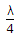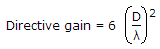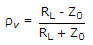# Electronics and Communication Engineering - Microwave Communication

Exercise : Microwave Communication - Section 2
6.
Consider the following statements
1. Impedance of Gunn diode is about tens of ohms.
2. Impedance of Impatt diode is a few ohms.
3. Impedance of Impatt diode are of the same order.
4. Impedance of Impatt diode is more than that of Gunn diode.
Which of the above statement are correct?
all
1 and 2 only
1, 2 and 3
1, 2 and 4
Explanation:

A Gunn diode uses GaAs which has a negative differential mobility, i.e., a decrease in carrier velocity with increase in electric field.

This effects is called transferred electron effect. The impedance of a Gunn diode is tens of ohms.

A Gunn diode oscillator has a resonant cavity, an arrangement to couple Gunn diode to cavity, biasing arrangement for Gunn diode and arrangement to couple RF power to load.

An Impatt diode has n+ - p - i - p + structure and is used with reverse bias.

It exhibits negative resistance and operates on the principle of avalanche breakdown. Impatt diode circuits are classified as broadly tunable circuit, low Q circuit and high Q circuit.

The impedance of Impatt diode is a few ohms. The word Impatt stands for Impact Avalanche Transit Time diode.

The features of Impatt diode oscillator are : Frequency 1 to 300 GHz, Power output (0.5 W to 5 W for single diode circuit and upto 40 W for combination of several diodes), efficiency about 20%.

Its applications include police radar systems, low power microwave transmitter etc.

7.
In high frequency circuits impedance matching can be done by
1. open circuited stubs
2. short circuited stubs
3.transformer
Which of the above correct?
1, 2 and 3
1 and 2 only
1 and 3 only
2 and 3 only
Explanation:

All the three can be used for impedance matching in high frequency circuits.

8.
The directive gain of a transmitting antenna is proportional to
its cross-sectional area
square of cross-sectional area
square root of cross-sectional area
cube root of cross-sectional area
Explanation:and is thus proportional to cross sectional area.

9.
In a loss line RL < Z0, then
Reflection coefficient is zero
Reflection coefficient is ∞
Reflection coefficient is negative
Reflection coefficient is positive
Explanation:Since RL < Z0, rv is negative.

10.

Assertion (A): A backward wave oscillator can be used as a sweep generator.

Reason (R): The frequency of oscillation of a backward wave oscillator can be changed by varying the voltage which controls the beam velocity.

Both A and R are correct and R is correct explanation of A
Both A and R are correct but R is not correct explanation of A
A is correct but R is wrong
A is wrong but R is correct
Explanation:

It is somewhat similar to TWT and can deliver microwave power over a wide frequency band.

It has an electron gun and a helix structure. However the interaction between electron beam and RF wave is different than in TWT.

The growing RF wave travels in opposite direction to the electron beam.

The frequency of wave can be changed by changing the voltage which controls the beam velocity.

Moreover the amplitude of oscillations can be decreased continuously to zero by changing the beam current.

It features are:

1. Frequency range - 1 GHz to 1000 GHz.

2. Power output - 10 mV to 150 mW (continuous wave) 250kW (pulsed).

It is used as signal source in transmitters and instruments.